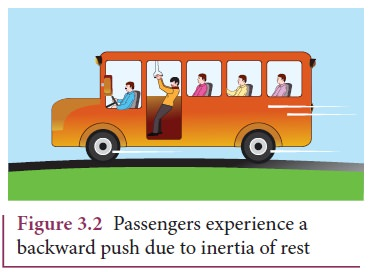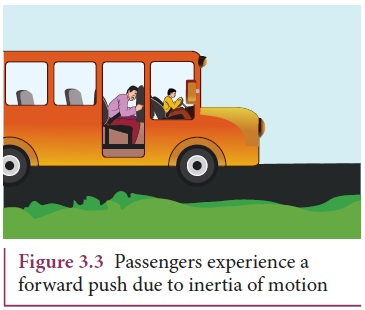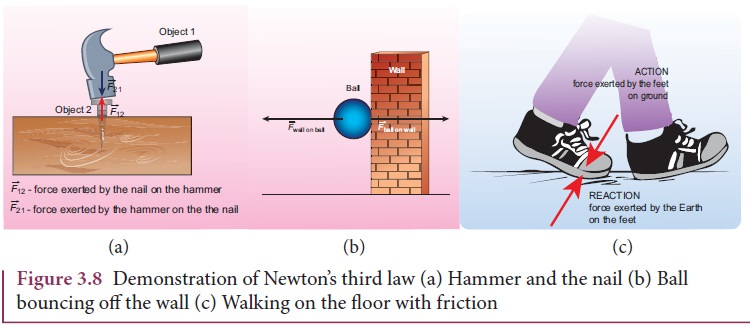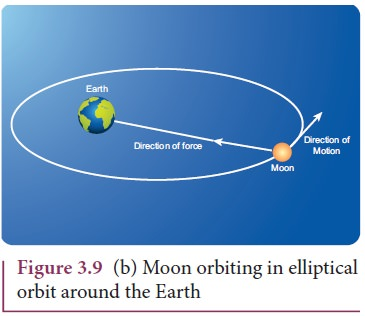Home | | Physics 11th std | Newton’s Law

# Newton’s Law

Newton analysed the views of Galileo, and other scientist like Kepler and Copernicus on motion and provided much deeper insights in the form of three laws.

NEWTON’S LAWS

Newton analysed the views of Galileo, and other scientist like Kepler and Copernicus on motion and provided much deeper insights in the form of three laws.

## Newton’s First Law

Every object continues to be in the state of rest or of uniform motion (constant velocity) unless there is external force acting on it.

This inability of objects to move on its own or change its state of motion is called inertia. Inertia means resistance to change its state. Depending on the circumstances, there can be three types of inertia.

1. Inertia of rest: When a stationary bus starts to move, the passengers experience a sudden backward push. Due to inertia, the body (of a passenger) will try tocontinue in the state of rest, while the bus moves forward. This appears as a backward push as shown in Figure 3.2. The inability of an object to change its state of rest is called inertia of rest.

2. Inertia of motion: When the bus is in motion, and if the brake is applied suddenly, passengers move forward and hit against the front seat. In this case, the bus comes to a stop, while the body (of a passenger) continues to move forward due to the property of inertia as shown in Figure 3.3. The inability of an object to change its state of uniform speed (constant speed) on its own is called inertia of motion.3. Inertia of direction: When a stone attached to a string is in whirling motion, and if the string is cut suddenly, the stone will not continue to move in circular motion but moves tangential to the circle as illustrated in Figure 3.4.This is because the body cannot change its direction of motion without any force acting on it. The inability of an object to change its direction of motion on its own is called inertia of direction.

When we say that an object is at rest or in motion with constant velocity, it has a meaning only if it is specified with respect to some reference frames. In physics, any motion has to be stated with respect to a reference frame. It is to be noted that Newton’s first law is valid only in certain special reference frames called inertial frames. In fact, Newton’s first law defines an inertial frame.

### Inertial Frames

If an object is free from all forces, then it moves with constant velocity or remains at rest when seen from inertial frames. Thus, there exists some special set of frames in which if an object experiences no force it moves with constant velocity or remains at rest. But how do we know whether an object is experiencing a force or not? All the objects in the Earth experience Earth’s gravitational force. In the ideal case, if an object is in deep space (very far away from any other object), then Newton’s first law will be certainly valid. Such deep space can be treated as an inertial frame. But practically it is not possible to reach such deep space and verify Newton’s first law.

For all practical purposes, we can treat Earth as an inertial frame because an object on the table in the laboratory appears to be at rest always. This object never picks up acceleration in the horizontal direction since no force acts on it in the horizontal direction. So the laboratory can be taken as an inertial frame for all physics experiments and calculations. For making these conclusions, we analyse only the horizontal motion of the object as there is no horizontal force that acts on it. We should not analyse the motion in vertical direction as the two forces (gravitational force in the downward direction and normal force in upward direction) that act on it makes the net force is zero in vertical direction. Newton’s first law deals with the motion of objects in the absence of any force and not the motion under zero net force. Suppose a train is moving with constant velocity with respect to an inertial frame, then an object at rest in the inertial frame (outside the train) appears to move with constant velocity with respect to the train (viewed from within the train). So the train can be treated as an inertial frame. All inertial frames are moving with constant velocity relative to each other. If an object appears to be at rest in one inertial frame, it may appear to move with constant velocity with respect to another inertial frame. For example, in Figure 3.5, the car is moving with uniform velocity v with respect to a person standing (at rest) on the ground. As the car is moving with constant velocity with respect to ground to the person is at rest on the ground, both frames (with respect to the car and to the ground) are inertial frames.Suppose an object remains at rest on a smooth table kept inside the train, and if the train suddenly accelerates (which we may not sense), the object appears to accelerate backwards even without any force acting on it. It is a clear violation of Newton’s first law as the object gets accelerated without being acted upon by a force. It implies that the train is not an inertial frame when it is accelerated. For example, Figure 3.6 shows that car 2 is a non-inertial frame since it moves with accelerationwith respect to the ground.These kinds of accelerated frames are called non-inertial frames. A rotating frame is also a non inertial frame since rotation requires acceleration. In this sense, Earth is not really an inertial frame since it has self-rotation and orbital motion. But these rotational effects of Earth can be ignored for the motion involved in our day-to-day life. For example, when an object is thrown, or the time period of a simple pendulum is measured in the physics laboratory, the Earth’s self-rotation has very negligible effect on it. In this sense, Earth can be treated as an inertial frame. But at the same time, to analyse the motion of satellites and wind patterns around the Earth, we cannot treat Earth as an inertial frame since its self-rotation has a strong influence on wind patterns and satellite motion.

## Newton’s Second Law

This law states that

The force acting on an object is equal to the rate of change of its momentumIn simple words, whenever the momentum of the body changes, there must be a force acting on it. The momentum of the object is defined as. In most cases, the mass of the object remains constant during the motion. In such cases, the above equation gets modified into a simpler formThe above equation conveys the fact that if there is an acceleration a on the body, then there must be a force acting on it. This implies that if there is a change in velocity, then there must be a force acting on the body. The force and acceleration are always in the same direction. Newton’s second law was a paradigm shift from Aristotle’s idea of motion. According to Newton, the force need not cause the motion but only a change in motion. It is to be noted that Newton’s second law is valid only in inertial frames. In non-inertial frames Newton’s second law cannot be used in this form. It requires some modification.

In the SI system of units, the unit of force is measured in newtons and it is denoted by symbol ‘N’.

One Newton is defined as the force which acts on 1 kg of mass to give an acceleration 1 m s-2 in the direction of the force.

### Aristotle vs. Newton’s approach on sliding object

Newton’s second law gives the correct explanation for the experiment on the inclined plane that was discussed in section 3.1. In normal cases, where friction is not negligible, once the object reaches the bottom of the inclined plane (Figure 3.1), it travels some distance and stops. Note that it stops because there is a frictional force acting in the direction opposite to its velocity. It is this frictional force that reduces the velocity of the object to zero and brings it to rest. As per Aristotle’s idea, as soon as the body reaches the bottom of the plane, it can travel only a small distance and stops because there is no force acting on the object. Essentially, he did not consider the frictional force acting on the object.Newton’s Third Law

Consider Figure 3.8(a) whenever an object 1 exerts a force on the object 2 (), then object 2 must also exert equal and opposite force on the object 1 (). These forces must lie along the line joining the two objects.Newton’s third law assures that the forces occur as equal and opposite pairs. An isolated force or a single force cannot exist in nature. Newton’s third law states that for every action there is an equal and opposite reaction. Here, action and reaction pair of forces do not act on the same body but on two different bodies. Any one of the forces can be called as an action force and the other the reaction force. Newton’s third law is valid in both inertial and non-inertial frames.These action-reaction forces are not cause and effect forces. It means that when the object 1 exerts force on the object 2, the object 2 exerts equal and opposite force on the body 1 at the same instant.

## Discussion on Newton’s Laws

1. Newton’s laws are vector laws. The equationis a vector equation and essentially it is equal to three scalar equations. In Cartesian coordinates, this equation can be written  asBy comparing both sides, the three scalar equations are

Fx = max The acceleration along the x direction depends only on the component of force acting along the x-direction.

Fy = may The acceleration along the y direction depends only on the component of force acting along the y-direction.

Fz = maz The acceleration along the z direction depends only on the component of force acting along the z-direction.

From the above equations, we can infer that the force acting along y direction cannot alter the acceleration along x direction. In the same way, Fz cannot afect ay and ax . This understanding is essential for solving problems.

2. The acceleration experienced by the body at time t depends on the force which acts on the body at that instant of time. It does not depend on the force which acted on the body before the time t. This can be expressed asAcceleration of the object does not depend on the previous history of the force. For example, when a spin bowler or a fast bowler throws the ball to the batsman, once the ball leaves the hand of the bowler, it experiences only gravitational force and air frictional force. The acceleration of the ball is independent of how the ball was bowled (with a lower or a higher speed).

3. In general, the direction of a force may be different from the direction of motion. Though in some cases, the object may move in the same direction as the direction of the force, it is not always true. A few examples are given below.

### Case 1: Force and motion in the same direction

When an apple falls towards the Earth, the direction of motion (direction of velocity) of the apple and that of force are in the same downward direction as shown in the Figure 3.9 (a).### Case 2: Force and motion not in the same direction

The Moon experiences a force towards the Earth. But it actually moves in elliptical orbit. In this case, the direction of the force is different from the direction of motion as shown in Figure 3.9 (b).### Case 3: Force and motion in opposite direction

If an object is thrown vertically upward, the direction of motion is upward, but gravitational force is downward as shown in the Figure 3.9 (c).Case 4: Zero net force, but there is motion When a raindrop gets detached from the cloud it experiences both downward gravitational force and upward air drag force. As it descends towards the Earth, the upward air drag force increases and after a certain time, the upward air drag force cancels the downward gravity. From then on the raindrop moves at constant velocity till it touches the surface of the Earth. Hence the raindrop comes with zero net force, therefore with zero acceleration but with non-zero terminal velocity. It is shown in the Figure 3.9 (d).4. If multiple forces .1 ,2 ,3 ...n act on the same body, then the total force (net ) is equivalent to the vectorial sum of the individual forces. Their net force provides the acceleration.In this case the direction of acceleration is in the direction of net force.5. Newton’s second law can also be written in the following form.

Since the acceleration is the second derivative of position vector of the bodyFrom this expression, we can infer that Newton’s second law is basically a second order ordinary differential equation and whenever the second derivative of position vector is not zero, there must be a force acting on the body.

6. If no force acts on the body then Newton’s second law,It implies that= constant. It is essentially Newton’s first law. It implies that the second law is consistent with the first law. However, it should not be thought of as the reduction of second law to the first when no force acts on the object. Newton’s first and second laws are independent laws. They can internally be consistent with each other but cannot be derived from each other.

7. Newton’s second law is cause and effect relation. Force is the cause and acceleration is the effect. Conventionally, the effect should be written on the left and cause on the right hand side of the equation. So the correct way of writing Newton’s second law isStudy Material, Lecturing Notes, Assignment, Reference, Wiki description explanation, brief detail
11th Physics : UNIT 3 : Laws of Motion : Newton’s Law |